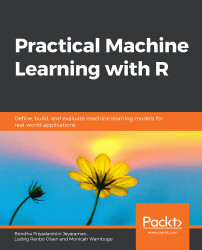•#### Practical Machine Learning with R#### Overview of this book

With huge amounts of data being generated every moment, businesses need applications that apply complex mathematical calculations to data repeatedly and at speed. With machine learning techniques and R, you can easily develop these kinds of applications in an efficient way. Practical Machine Learning with R begins by helping you grasp the basics of machine learning methods, while also highlighting how and why they work. You will understand how to get these algorithms to work in practice, rather than focusing on mathematical derivations. As you progress from one chapter to another, you will gain hands-on experience of building a machine learning solution in R. Next, using R packages such as rpart, random forest, and multiple imputation by chained equations (MICE), you will learn to implement algorithms including neural net classifier, decision trees, and linear and non-linear regression. As you progress through the book, you’ll delve into various machine learning techniques for both supervised and unsupervised learning approaches. In addition to this, you’ll gain insights into partitioning the datasets and mechanisms to evaluate the results from each model and be able to compare them. By the end of this book, you will have gained expertise in solving your business problems, starting by forming a good problem statement, selecting the most appropriate model to solve your problem, and then ensuring that you do not overtrain it.Free Chapter
An Introduction to Machine LearningData Cleaning and Pre-processingFeature EngineeringIntroduction to neuralnet and Evaluation MethodsLinear and Logistic Regression ModelsUnsupervised LearningAppendix## Model Selection

Now that we know how to train a classifier, we will revise how to evaluate and choose between models. First, we will cover four common metrics: Accuracy, Precision, Recall, and F1, and then we will discuss cross-validation as a good tool for model comparison.

### Evaluation Metrics

We use a confusion matrix to split the predictions into True Positives, False Positives, True Negatives, and False Negatives. In our case, True Positives are the applicants that were correctly identified as being "Good," False Positives are the applicants that were incorrectly identified as being "Good," True Negatives are the applicants that were correctly identified as "Bad," while False Negatives are the applicants that were incorrectly identified as "Bad."

###### Figure 4.4: Confusion matrix

In Figure 4.4, the rows are the true classes, while the columns are the predicted classes. If the true class is Positive and the model predicts Positive, that prediction...